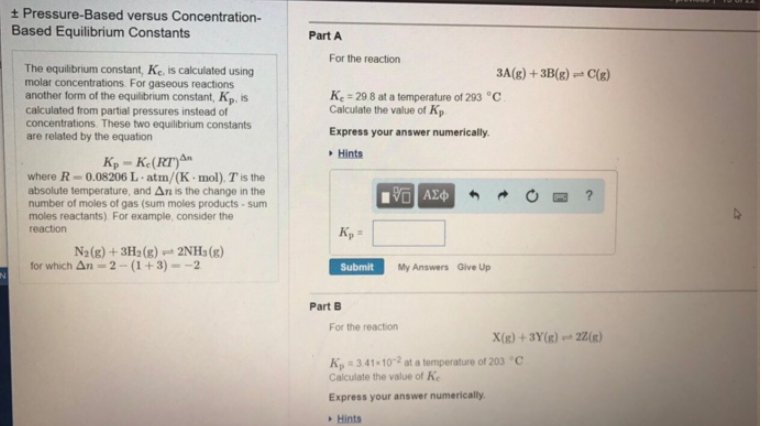# Problem: The equilibrium constant, Kc, is calculated using molar concentrations. For gaseous reactions another form of the equilibrium constant, Kp, is calculated from partial pressures instead of concentrations. These two equilibrium constants are related by the equation Kp = Kc (RT)Δn where R = 0.08206 L•atm/(K•mol), T is the absolute temperature, and Δn is the change in the number of moles of gas (sum moles product - sum moles reactants). For example, consider the reaction N2(g) + 3H2(g) ⇌ 2NH3(g)  for which Δn = 2 - (1 + 3) = -2 Part A. For the reaction 3A(g) + 3B(g) ⇌ C(g) Kc = 29.8 at a temperature of 293°C. Calculate the value of Kp. Express your answer numerically. Part B. For the reaction X(g) + 3Y(g) ⇌ 2Z(g) Kp = 3.41 x 10-2 at a temperature of 203°C. Calculate the value of Kc. Express your answer numerically.

###### FREE Expert Solution
85% (320 ratings)###### Problem Details

The equilibrium constant, Kc, is calculated using molar concentrations. For gaseous reactions another form of the equilibrium constant, Kp, is calculated from partial pressures instead of concentrations. These two equilibrium constants are related by the equation

Kp = Kc (RT)Δn

where R = 0.08206 L•atm/(K•mol), T is the absolute temperature, and Δn is the change in the number of moles of gas (sum moles product - sum moles reactants). For example, consider the reaction

N2(g) + 3H2(g) ⇌ 2NH3(g)

for which Δn = 2 - (1 + 3) = -2

Part A.

For the reaction

3A(g) + 3B(g) ⇌ C(g)

Kc = 29.8 at a temperature of 293°C. Calculate the value of Kp. Express your answer numerically.

Part B.

For the reaction

X(g) + 3Y(g) ⇌ 2Z(g)

Kp = 3.41 x 10-2 at a temperature of 203°C. Calculate the value of Kc. Express your answer numerically.